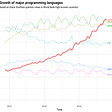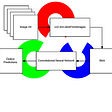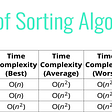# Big O — Time Complexity Graph Simplified

When I began my journey in learning algorithms to become a better coder, this was the first big lesson — “Big O”. The name was daunting enough but after watching several videos and seeing in action how important Big O is, I wanted to explain it in one blog.

So Big O is all about “time complexity” (will be omitting space complexity in this blog). It gives us a numeric value (O notation) of how well our code performs, especially in a worst-case scenario of our input being a very large number or an array.

The problem with measuring how “long” it takes to complete a function is that computers will not have a precise amount of time for how fast a function completes. Even the same computer will give you different speeds that a function would complete. So instead of counting the seconds it takes to complete, we will count the number of operations the computer has to complete.

With Big O, we don’t care about the details of the function, we only care about the broad trends.

Big O notation is how we get our numeric value of how our code performs.

In the graph above, the X-axis represents the input value as it gets longer and the Y-axis represents the amount of time elapsed to perform the algorithm (function).

Functions with an O notation of O(1) will complete the fastest because it doesn’t matter how large the input value is, the same amount of time will elapse to complete the function. This is constant time complexity. The O notation values in green are considered great because the number of inputs does not change the time it takes to complete the function.

Function with an O notation of O(n), in yellow, will complete at an okay time. The time it takes to complete the function depends on the number of inputs. It is about a 1:1 (one-to-one) linear ratio in time. The more inputs, the longer it will take in proportion to the inputs. These are not the greatest but they are considerably better than the ones in red.

The O notations in red, such as O(N²) and O(N log N) are very bad. They have a quadratic ratio or worse. The inputs take a long time to complete the function and the larger the input, the longer time it will take. This is much different than the O(n) notation because it’s not linear. If the input here is 2, then the time will take 4 times as long to complete compared to an O(n) notation.

Big O gives us a high-level understanding of the time complexity of a function (algorithm). Precision does not matter with Big O. Only the general trend matters whether it’s constant, linear, quadratic, or logarithmic.

--

--

--

## More from Ajak Cyer

Software Engineering Student at Flatiron NYC. Learning day by day 💻

Love podcasts or audiobooks? Learn on the go with our new app.

## Data Pipeline Orchestration on Steroids: Apache Airflow Tutorial, Part 1## HNGi8 Internship## How to make a simple API with python and bottle## Choosing a PostgreSQL client## Writing Professional Python Code## Ajak Cyer

Software Engineering Student at Flatiron NYC. Learning day by day 💻

## Solving LeetCode #1 Two Sum Using Javascript In O(n)## TECH EYE## weekly updates: learning algos & JS backend[3/10/2022]